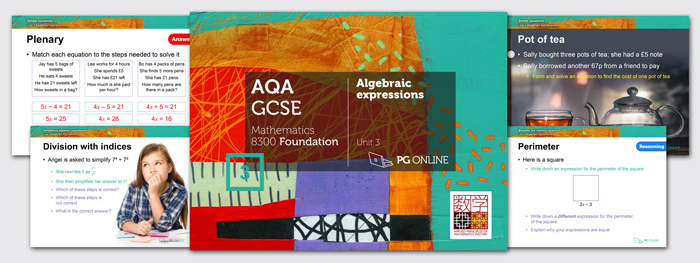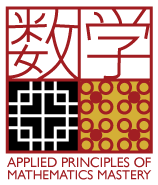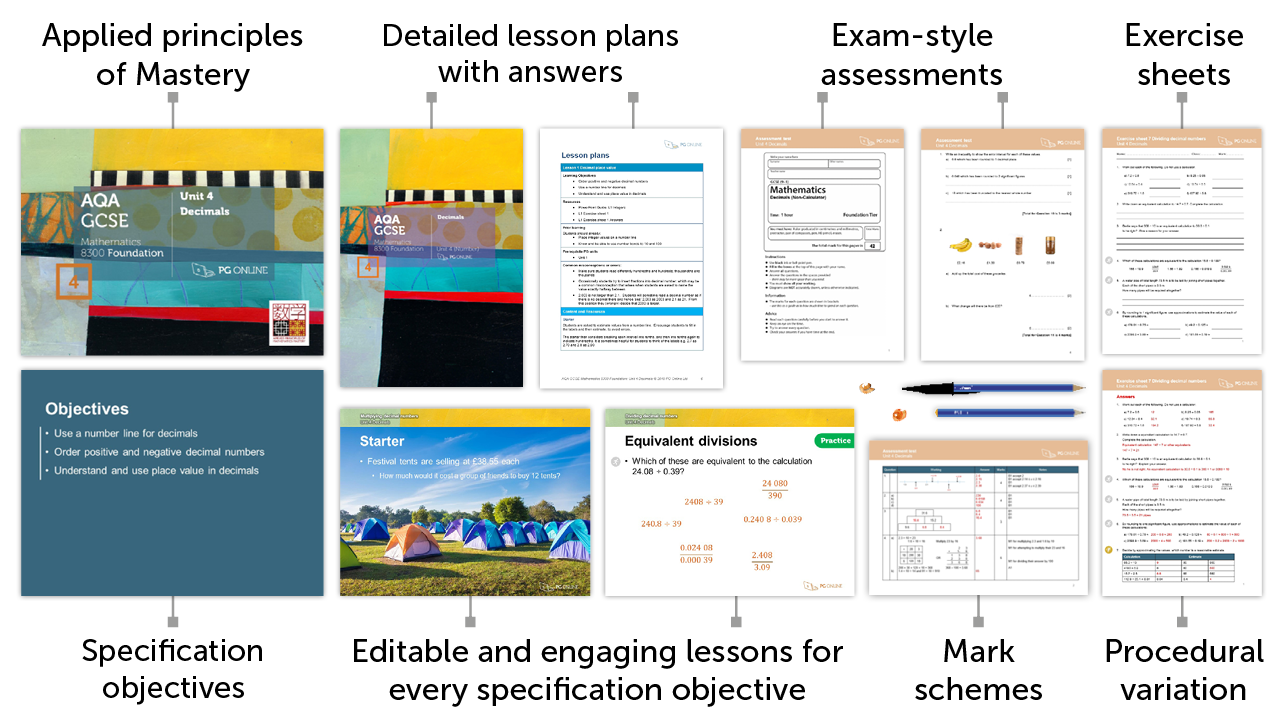# Mastery for GCSE AQA

### £75.00 + VATDiscounted rate from £15+

3

## Inside the Unit...### Areas Covered

• Lesson 1: Algebraic notation
• Lesson 2: Expressions as functions
• Lesson 3: Algebraic expressions
• Lesson 4: Simple equations
• Lesson 5: Simple formula
• Lesson 6: Brackets and common factors
• Lesson 7: Powers and roots
• Unit assessment

There are 7 exercise sheets and an examination-style assessment test, each with answers included in this unit.

## Mathematics Mastery

Our resources apply the principles of Mastery throughout, creating a rich and deep learning experience. Find out more about Mastery with our quick guide.## Summary

This unit returns to the basics of algebra to ensure fluency in the bedrock of algebraic techniques. Algebraic notation is considered; the representation of an unknown by a letter, the four operations in algebra and index numbers. Algebraic expressions are introduced and used to represent information given in a context. The substitution of numbers into algebraic expressions is dealt with, including into known and given scientific formula. Algebraic expressions are manipulated and simplified by collecting like terms, cancelling terms, multiplying and dividing terms, expanding brackets and factorising. Algebraic terms involving indices and surds are met and manipulated using the laws of indices. The unit ensures students understand key terminology relating to algebra such as expression, equation and formulae, and continues to deal with the formation and solving of simple equations. Rearranging of simple formulae is considered including changing the subject of a formula involving indices.

## What's included in the toolkit?The GCSE units have been written to satisfy the specification for the AQA 8300 Maths GCSE. Each unit contains:

• PowerPoint slides for each component
• Detailed lesson plans
• Learning objectives and outcomes
• Worksheets and homework activities with answers
• End-of-unit, exam-style assessment test with answers
• Other material and links to online resources

## What people say...

Thank you – I have found PG Online resources extremely useful.

The content was outstanding at supporting Higher level students.

Bethan Atkins. Science teacher, Lutterworth College

## Inside the Unit...

### Areas Covered

• Lesson 1: Algebraic notation
• Lesson 2: Expressions as functions
• Lesson 3: Algebraic expressions
• Lesson 4: Simple equations
• Lesson 5: Simple formula
• Lesson 6: Brackets and common factors
• Lesson 7: Powers and roots
• Unit assessment

There are 7 exercise sheets and an examination-style assessment test, each with answers included in this unit.

## Mathematics Mastery

Our resources apply the principles of Mastery throughout, creating a rich and deep learning experience. Find out more about Mastery with our quick guide.• ### How to order

1. Add individual units to a draft order or download a blank order form below to complete manually

2. Using a draft order you can either:

2. Email us your complete order
3. Create a PDF
(to fax or email at a later date)

• ### Unit Pricing and Discounts

Each unit is individually priced for LIFE. Please view each unit for detail.

Discounts are cumulative based on the total of units licenced to your department.

All prices subject to further discounts and VAT.

There are no annual renewal fees.

 2-5 units 10% discount 6-11 units 15% discount 12-23 units 20% discount 24+ units 25% discount